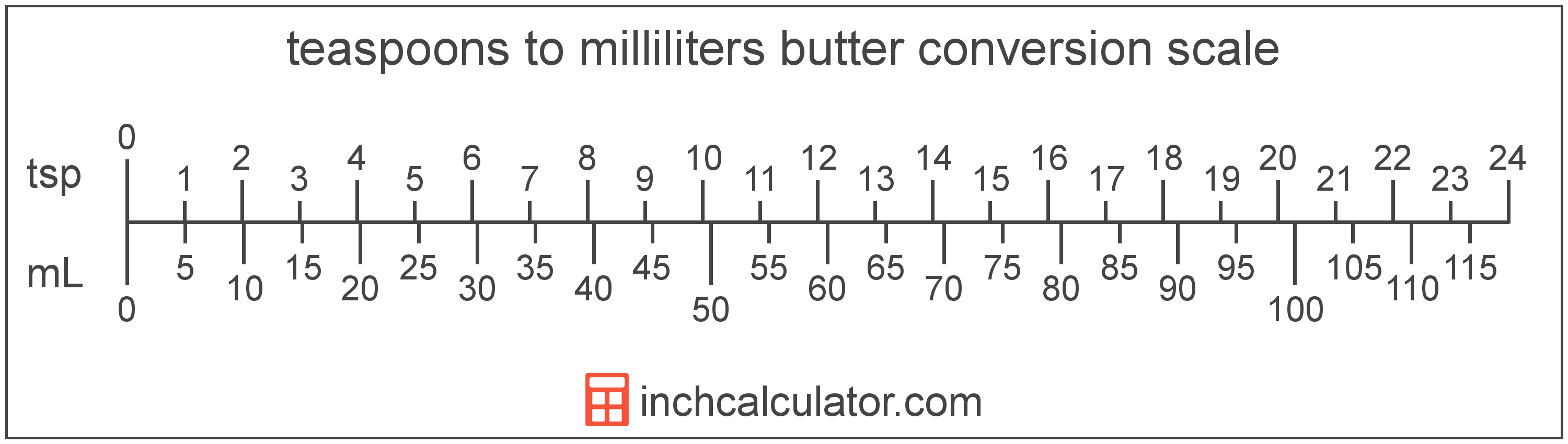# Convert Teaspoons of Butter to Milliliters

Enter the butter in teaspoons below to get the value converted to milliliters.

Results in Milliliters:1 tsp = 4.928922 mL

Do you want to convert milliliters to teaspoons?

## How to Convert Teaspoons to Milliliters

To convert a measurement in teaspoons to a measurement in milliliters, multiply the butter by the following conversion ratio: 4.928922 milliliters/teaspoon.

Since one teaspoon of butter is equal to 4.928922 milliliters, you can use this simple formula to convert:

milliliters = teaspoons × 4.928922

The butter in milliliters is equal to the butter in teaspoons multiplied by 4.928922.

For example, here's how to convert 5 teaspoons to milliliters using the formula above.
milliliters = (5 tsp × 4.928922) = 24.644608 mL### How Many Milliliters Are in a Teaspoon of Butter?

There are 4.928922 milliliters in a teaspoon of butter, which is why we use this value in the formula above.

1 tsp = 4.928922 mL

## What Is a Teaspoon?

One teaspoon of butter is equal to 1/3 of a tablespoon or about 4.7 grams.

The teaspoon is a US customary unit of volume. Teaspoons can be abbreviated as tsp, and are also sometimes abbreviated as t, ts, or tspn. For example, 1 teaspoon can be written as 1 tsp, 1 t, 1 ts, or 1 tspn.

## What Is a Milliliter?

Butter is sometimes measured by volume in milliliters. One stick of butter contains about 118.3 milliliters.

The milliliter is an SI unit of volume in the metric system. A milliliter is sometimes also referred to as a millilitre. Milliliters can be abbreviated as mL; for example, 1 milliliter can be written as 1 mL.

## Teaspoon to Milliliter Conversion Table

Table showing various teaspoon measurements converted to milliliters.
Teaspoons Milliliters
1 tsp 4.9289 mL
2 tsp 9.8578 mL
3 tsp 14.79 mL
4 tsp 19.72 mL
5 tsp 24.64 mL
6 tsp 29.57 mL
7 tsp 34.5 mL
8 tsp 39.43 mL
9 tsp 44.36 mL
10 tsp 49.29 mL
11 tsp 54.22 mL
12 tsp 59.15 mL
13 tsp 64.08 mL
14 tsp 69 mL
15 tsp 73.93 mL
16 tsp 78.86 mL
17 tsp 83.79 mL
18 tsp 88.72 mL
19 tsp 93.65 mL
20 tsp 98.58 mL
21 tsp 103.51 mL
22 tsp 108.44 mL
23 tsp 113.37 mL
24 tsp 118.29 mL
25 tsp 123.22 mL
26 tsp 128.15 mL
27 tsp 133.08 mL
28 tsp 138.01 mL
29 tsp 142.94 mL
30 tsp 147.87 mL
31 tsp 152.8 mL
32 tsp 157.73 mL
33 tsp 162.65 mL
34 tsp 167.58 mL
35 tsp 172.51 mL
36 tsp 177.44 mL
37 tsp 182.37 mL
38 tsp 187.3 mL
39 tsp 192.23 mL
40 tsp 197.16 mL